# Solution assignment 05 Area and volume

### Assignment 5

Calculate the area which is enclosed by the circle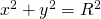, that is a circle with center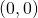and radius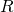.

### Solution

The area of the circle can be calculated by first calculating the area of the circle in the first quadrant and multiply it by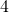. The function in the first quadrant is: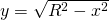and thus we have to calculate: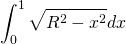First we apply the substitution method: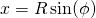, with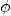ranging from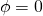to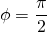which results in: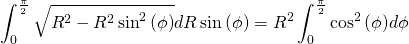Now we use a formula from trigonometry: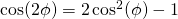and thus: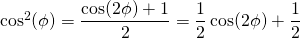Then the integral becomes: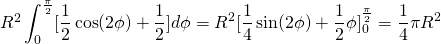The area of the whole circle is thus. This is the result we already knew.